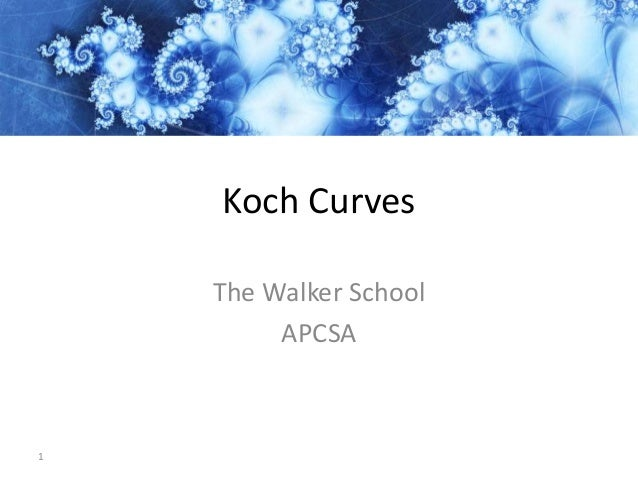Successfully reported this slideshow.
We use your LinkedIn profile and activity data to personalize ads and to show you more relevant ads. You can change your ad preferences anytime.Upcoming SlideShare
×

# Java Koch Curves

2,220 views

Published on

This slide deck explains how to create landmasses from Koch Curves using recursion. The programming language used is Java.

Published in: Education
• Full Name
Comment goes here.

Are you sure you want to Yes No
Your message goes here• Be the first to comment

• Be the first to like this

### Java Koch Curves

1. 1. Koch Curves The Walker School APCSA 1
2. 2. 2 Koch Curve
3. 3. 3 Depth 1 1 line segment
4. 4. 4 Depth 2 Contains 4 depth-1 curves (4 line segments)
5. 5. 5 Depth 3 Contains 4 depth-2 curves (16 line segments)
6. 6. 6 Depth 4 Contains 4 depth-3 curves (64 line segments)
7. 7. 7 Depth 5 Contains 4 depth-4 curves (256 line segments)
8. 8. 8 Pseudocode Algorithm To make a Koch Curve: • Draw a straight line if depth is zero; otherwise draw four smaller Koch Curves.
9. 9. Koch Curve Start with a line.
10. 10. Line Segment Transformation (x1,y1) (x2,y2) (x3,y3) (x4,y4) (x5,y5) (x1,y1) (x5,y5)
11. 11. Segment Calculations
12. 12. Calculating the Projection l l x = ½l y = √3/2l m2 + (½ l)2 = l2 m2 + ¼ l2 = l2 √m2 = √¾ l2 m = √3/2l * 1/3 = √3l/6 1/3 length of each iteration
13. 13. Other Geometric Shapes?
14. 14. Koch Snowflake Start with a triangle.
15. 15. Triangles Need 3 Calls to drawFractal() Call the method drawFractal() for each side of the triangle. You need to establish variables for the x,y coordinates for the line on each side of the base triangle.
16. 16. What aspects can you change?
17. 17. Aspects • Ratio Expression • Shape of the Triangle • Shape of the Base – Square, Pentagon, Trapazoid • Tessellations • Color
18. 18. Ratio Change to Projection How does the projection change when you change the ratio?
19. 19. Change Ratio through Expressions
20. 20. Change Base to Koch Square Start with a square.
21. 21. Calculates the Tip of the Projection How does the projection change when you change the ratio?
22. 22. Square Needs 4 Calls to drawFractal()
23. 23. How do we get curves that look like landmasses?
24. 24. 24 Fractals in Nature Coastlines
25. 25. 25 Fractals in Nature Coastlines
26. 26. 26 Fractals in Nature Coastlines
27. 27. 27 Fractals in Nature Coastlines
28. 28. 28 Fractals in Nature Coastlines
29. 29. 29 Fractals in Nature Coastlines
30. 30. 30 Fractals in Nature Coastlines
31. 31. Use the Random Class
32. 32. Add Randomness to the Ration Generates a random number between 3 and 10 for the denominator of the ratio.
33. 33. 3 Calls to the Method drawFractal()
34. 34. 6 Recursive Calls Approximates Landmass
35. 35. Examples for Inspiration
36. 36. Koch Snowflake Tessellations You can tessellate Koch snowflakes to create pattern on a canvas.
37. 37. Cesaro Curves Cesaro curves are variations on a Koch snowflake.
38. 38. Koch Curve (4/3n or log 4/log 3 = approx. 1.26) https://www.behance.net/gallery/720515/Worlds-Largest-Fractal-Vectors
39. 39. Contact If you want the Java code for some of the basic Koch Curves you can contact me at: Thomas Cooper The Walker School Marietta, GA 30062 Website: http://www.thewalkerschool.org Email: thomas.cooper@thewalkerschool.org